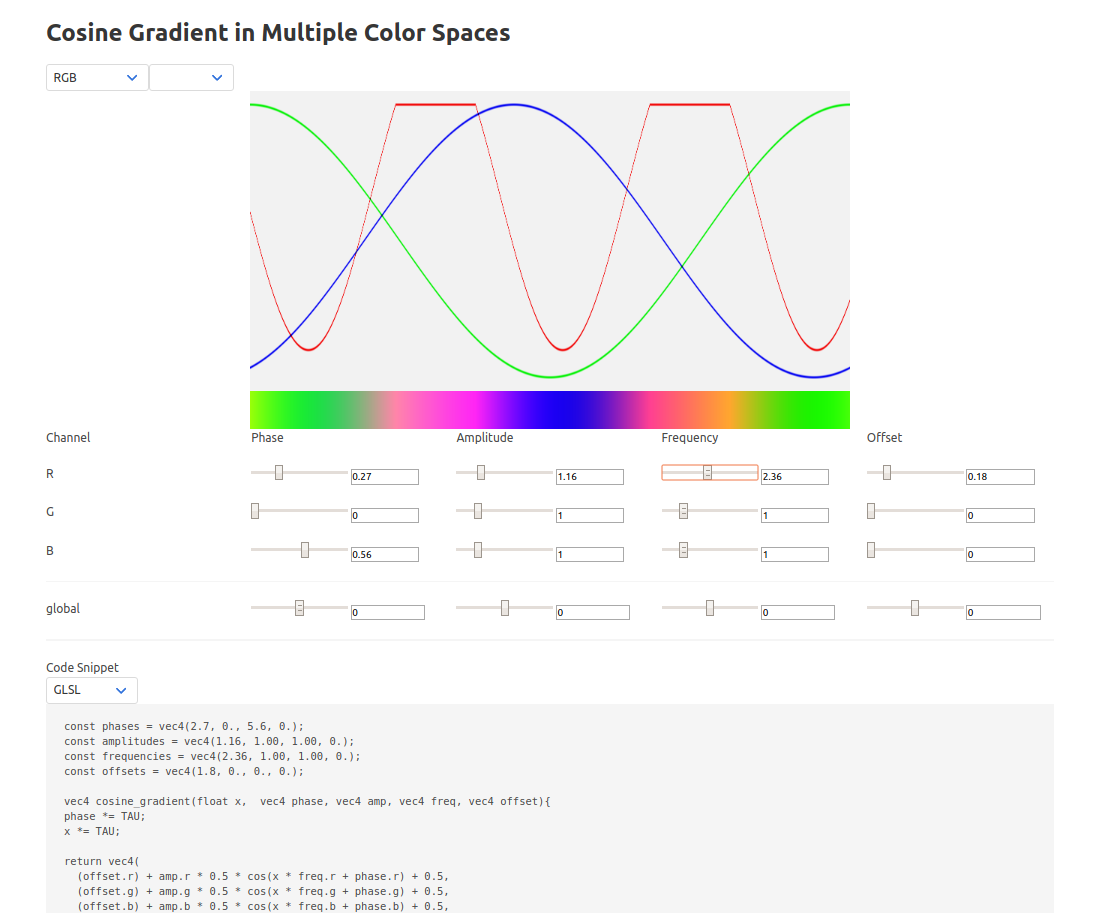# Grad

This is a cosine gradient generator implemented with Elm 0.19. The idea of a cosine gradient is pretty famous among creative coders, each RGB component is modulated with a cosine function.

$r = A_r\\cos{(f_r t + p_r)} \\\\ g = A_g\\cos{(f_g t + p_g)} \\\\ b = A_b\\cos{(f_b t + p_b)} \\\\ color = (r,g,b)$

This results in a surprising variety of color gradients which can easily be modulated in the range [0, 2π). In this experiment, I tried implementing other color spaces to see what other kinds of gradients could be made. There is also a simple code snippet for GLSL and Unity which can be copy pasted for ease of use.